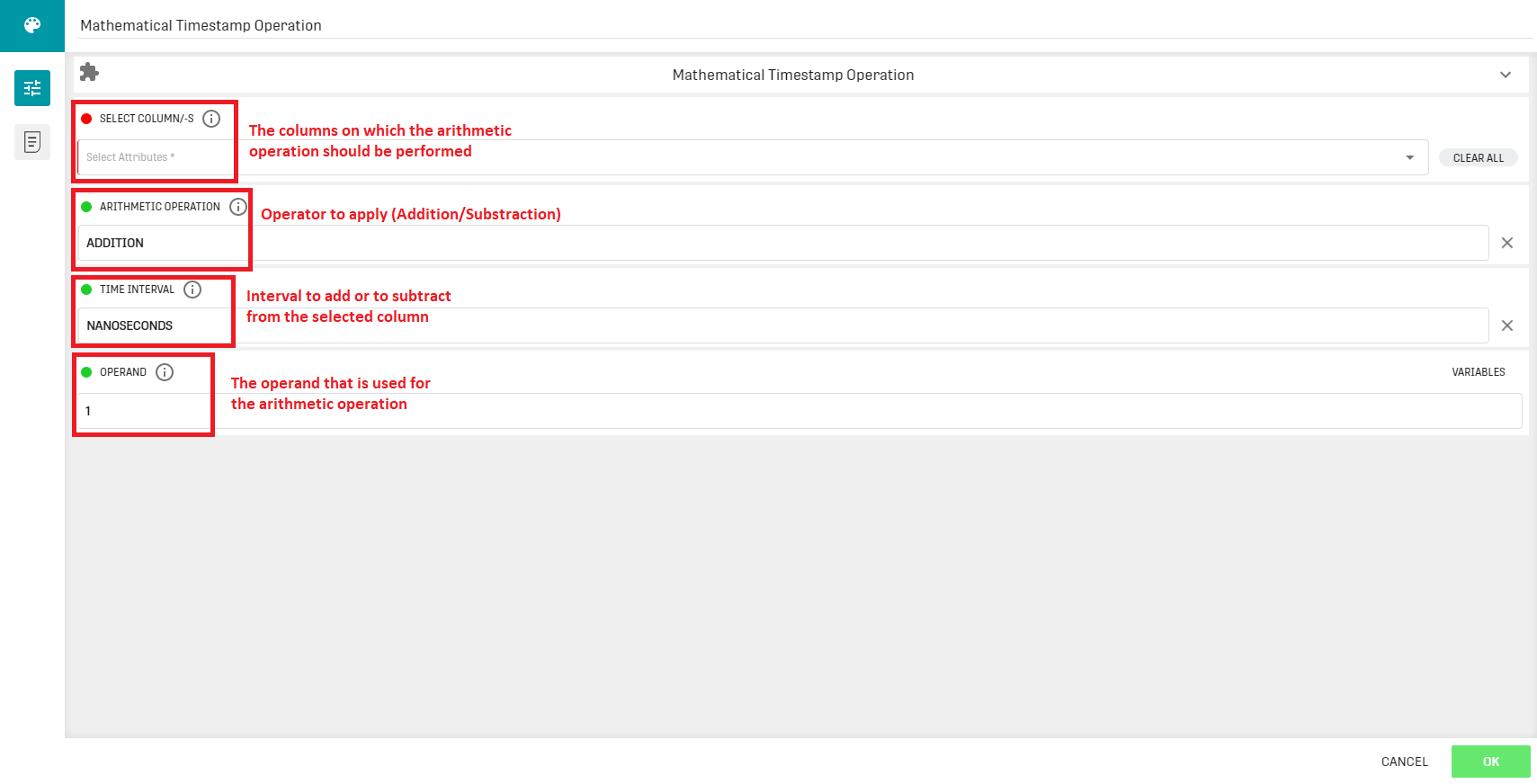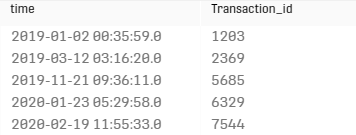# Overview

The Mathematical Timestamp Operation Processor adds or subtracts a user specified time value from one or several selected columns.

# Input

The processor requires a input dataset that contains at least one timestamp column.

# ConfigurationThe choices for the time interval are: Days, Hours, Minutes, Seconds, Milliseconds, Microseconds, Nanoseconds.
This processor can be useful when the time variable must be very precise, given that fractions of a second cannot be manually set in a datetime input variable.

# Output

The processor forwards the input dataset with the altered timestamp column(s).

# Example

## ResultThe selected time column is updated with the new values. The second column is forwarded as it is.

Note that a precision digit is appended to the previous time format. The new format supports fractions of a second (milliseconds, nanoseconds). Also note that adding or subtracting a time interval such as 00:15:30 (15 minutes and 30 seconds) requires two processors.

# Related Articles

Mathematical Operation SC Processor

Extended Mathematical Operation Processor

Mathematical Column Operation Processor